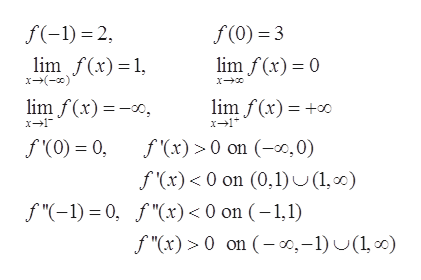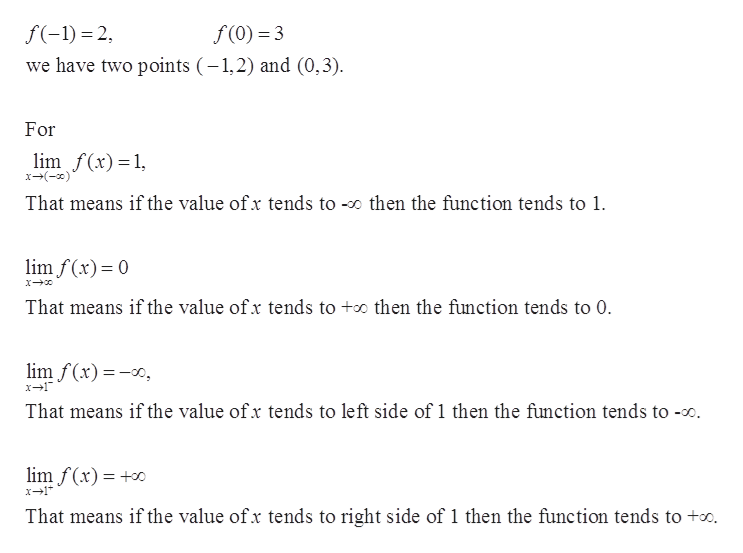# Sketch the graph of y = f(x), given the following: • f(−1) = 2; f(0) = 3; lim x→−∞ f(x) = 1; lim x→∞ f(x) = 0; lim x→1−f(x) = −∞; lim x→1+f(x) =+∞ • f'(0) = 0; f0(x) > 0 for x ∈ (−∞,0); f'(x) < 0 for x ∈ (0,1)∪(1,∞) • f"(−1) = 0; f"(x) < 0 for x ∈ (−1,1); f"(x) > 0 for x ∈ (−∞,−1)∪(1,∞)

Question
12 views

Sketch the graph of y = f(x), given the following: • f(−1) = 2; f(0) = 3; lim x→−∞ f(x) = 1; lim x→∞ f(x) = 0; lim x→1−
f(x) = −∞; lim x→1+
f(x) =
+∞ • f'(0) = 0; f0(x) > 0 for x ∈ (−∞,0); f'(x) < 0 for x ∈ (0,1)∪(1,∞) • f"(−1) = 0; f"(x) < 0 for x ∈ (−1,1); f"(x) > 0 for x ∈ (−∞,−1)∪(1,∞)

check_circle

star
star
star
star
star
1 Rating
Step 1

To sketch the graph of function y=f(x).

Step 2

Given conditions:help_outlineImage Transcriptionclosef(-1) = 2, f(0) = 3 lim f(x)=1, x-(-x) lim f(x) = 0 lim f(x) =-0, lim f(x) = +o x-1* f '(0) = 0, f (x) > 0 on (-o,0) f '(x)< 0 on (0,1) U(1, 00) f "(-1) = 0, f "(x) < 0 on (-1,1) f "(x) > 0 on (– 0,–1) U(1, 00) fullscreen
Step 3

For first cond...help_outlineImage Transcriptionclosef(-1) = 2, f (0) = 3 we have two points (-1,2) and (0,3). For lim f(x)=1, x-(-x)* That means if the value of x tends to -o then the function tends to 1. lim f(x)= 0 That means if the value of x tends to +o then the function tends to 0. lim f(x) =-x, x-1" That means if the value of x tends to left side of 1 then the function tends to -o. lim f (x) = +0 x-1* That means if the value of x tends to right side of 1 then the function tends to +o. fullscreen

### Want to see the full answer?

See Solution

#### Want to see this answer and more?

Solutions are written by subject experts who are available 24/7. Questions are typically answered within 1 hour.*

See Solution
*Response times may vary by subject and question.
Tagged in

### Functions